Next: An example fixed-step RK4 Up: Integration of ODEs Previous: Numerical instabilities

## Runge-Kutta methods

There are two main reasons why Euler's method is not generally used in scientific computing. Firstly, the truncation error per step associated with this method is far larger than those associated with other, more advanced, methods (for a given value of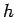). Secondly, Euler's method is too prone to numerical instabilities.

The methods most commonly employed by scientists to integrate o.d.e.s were first developed by the German mathematicians C.D.T. Runge and M.W. Kutta in the latter half of the nineteenth century.14The basic reasoning behind so-called Runge-Kutta methods is outlined in the following.

The main reason that Euler's method has such a large truncation error per step is that in evolving the solution from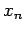to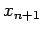the method only evaluates derivatives at the beginning of the interval: i.e., at. The method is, therefore, very asymmetric with respect to the beginning and the end of the interval. We can construct a more symmetric integration method by making an Euler-like trial step to the midpoint of the interval, and then using the values of bothandat the midpoint to make the real step across the interval. To be more exact,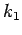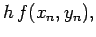(19)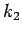(20)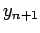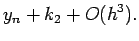(21)

As indicated in the error term, this symmetrization cancels out the first-order error, making the method second-order. In fact, the above method is generally known as a second-order Runge-Kutta method. Euler's method can be thought of as a first-order Runge-Kutta method.

Of course, there is no need to stop at a second-order method. By using two trial steps per interval, it is possible to cancel out both the first and second-order error terms, and, thereby, construct a third-order Runge-Kutta method. Likewise, three trial steps per interval yield a fourth-order method, and so on.15

The general expression for the total error,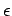, associated with integrating our o.d.e. over an-interval of order unity using anth-order Runge-Kutta method is approximately(22)

Here, the first term corresponds to round-off error, whereas the second term represents truncation error. The minimum practical step-length,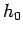, and the minimum error,, take the values(23)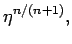(24)

respectively. In Tab. 1, these values are tabulated againstusing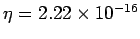(the value appropriate to double precision arithmetic on IBM-PC clones). It can be seen thatincreases anddecreases asgets larger. However, the relative change in these quantities becomes progressively less dramatic asincreases.

Table 1: The minimum practical step-length,, and minimum error,, for anth-order Runge-Kutta method integrating over a finite interval using double precision arithmetic on an IBM-PC clone.1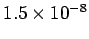2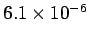3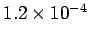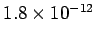4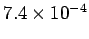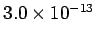5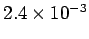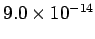In the majority of cases, the limiting factor when numerically integrating an o.d.e. is not round-off error, but rather the computational effort involved in calculating the function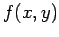. Note that, in general, anth-order Runge-Kutta method requiresevaluations of this function per step. It can easily be appreciated that asis increased a point is quickly reached beyond which any benefits associated with the increased accuracy of a higher order method are more than offset by the computational cost'' involved in the necessary additional evaluation ofper step. Although there is no hard and fast general rule, in most problems encountered in computational physics this point corresponds to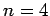. In other words, in most situations of interest a fourth-order Runge Kutta integration method represents an appropriate compromise between the competing requirements of a low truncation error per step and a low computational cost per step.

The standard fourth-order Runge-Kutta method takes the form:(25)(26)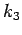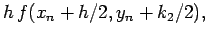(27)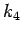(28)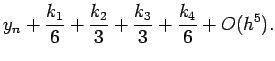(29)

This is the method which we shall use, throughout this course, to integrate first-order o.d.e.s. The generalization of this method to deal with systems of coupled first-order o.d.e.s is (hopefully) fairly obvious.Next: An example fixed-step RK4 Up: Integration of ODEs Previous: Numerical instabilities
Richard Fitzpatrick 2006-03-29